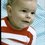# Brilliant standards

This week two of the math questions were wrong. Ons was "Sreejato's ordered pairs", the other one was "Strings with brackets". Sreejato's problem as it was first posed was simply impossible (the assumption f(n)=12 was impossible, it always had to be odd). And yet there were 60 people orso that had solved the problem...by guessing the correct question! Two days later the question changed to f(n)=13. The second question was actually possible as it was first posed, but then it said my answer was wrong. Then again I saw this question change (even twice) during the week. I don't think the idea of the site is for people to guess correct questions. It would be sad if people get annoyed and started dropping out.

-michaelNote by Michael May
6 years, 5 months ago

This discussion board is a place to discuss our Daily Challenges and the math and science related to those challenges. Explanations are more than just a solution — they should explain the steps and thinking strategies that you used to obtain the solution. Comments should further the discussion of math and science.

When posting on Brilliant:

• Use the emojis to react to an explanation, whether you're congratulating a job well done , or just really confused .
• Ask specific questions about the challenge or the steps in somebody's explanation. Well-posed questions can add a lot to the discussion, but posting "I don't understand!" doesn't help anyone.
• Try to contribute something new to the discussion, whether it is an extension, generalization or other idea related to the challenge.

MarkdownAppears as
*italics* or _italics_ italics
**bold** or __bold__ bold
- bulleted- list
• bulleted
• list
1. numbered2. list
1. numbered
2. list
Note: you must add a full line of space before and after lists for them to show up correctly
paragraph 1paragraph 2

paragraph 1

paragraph 2

[example link](https://brilliant.org)example link
> This is a quote
This is a quote
    # I indented these lines
# 4 spaces, and now they show
# up as a code block.

print "hello world"
# I indented these lines
# 4 spaces, and now they show
# up as a code block.

print "hello world"
MathAppears as
Remember to wrap math in $$ ... $$ or $ ... $ to ensure proper formatting.
2 \times 3 $2 \times 3$
2^{34} $2^{34}$
a_{i-1} $a_{i-1}$
\frac{2}{3} $\frac{2}{3}$
\sqrt{2} $\sqrt{2}$
\sum_{i=1}^3 $\sum_{i=1}^3$
\sin \theta $\sin \theta$
\boxed{123} $\boxed{123}$

Sort by:

The question "Sreejato's ordered pairs" was indeed wrong, and we had a typo in the question.

The question "Strings with brackets" was posed correctly. As some students were confused with the exact meaning of the last phrase, I decided to write out the condition explicitly. The meaning of the question has not been changed in the different versions. If your answer was marked wrong, you must have taken an incorrect interpretation of the condition.

Staff - 6 years, 5 months ago

Initially the question said "for k=1,2, we see k('s before k)'s?" I don't really want to comment on ongoing problems, but for k=2 it says we see 2 ('s before 2 )'s, which gives a little less solutions as to how the question is posed now. "The first ( occurs before the first )" and "the second ( occurs before the second )?" Or maybe I'm just still confused about the original question. Anyway, there is no confusion possible with how it's stated now, so that's good.

-michael

- 6 years, 5 months ago

As I have mentioned in the discussions thread of the solution, the error in the question was a typing error. In my original question, I had asked for the smallest prime power $N$ satisfying $f(N)= 7$. It was changed to $12$ when it was published, which is most possibly a typo.

- 6 years, 5 months ago

Don't you mean $f(N)=13$?

- 6 years, 5 months ago

In his original version, he gave $f(N) = 7$, which had an answer of 8. I felt that this was too close 7 which would make it lucky guess. Hence, I decided to change the question to $f(N) = 13$ instead. At this point, I introduced a typo and wrote $f(N) = 12$ instead. This error slipped through our proof-reading.

Staff - 6 years, 5 months ago